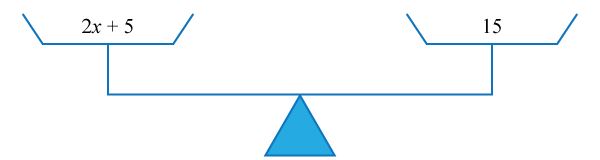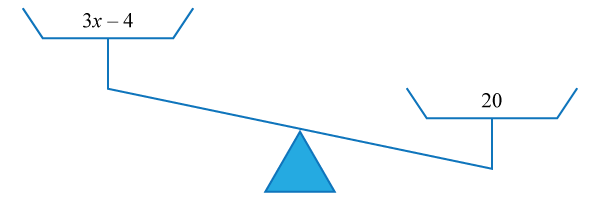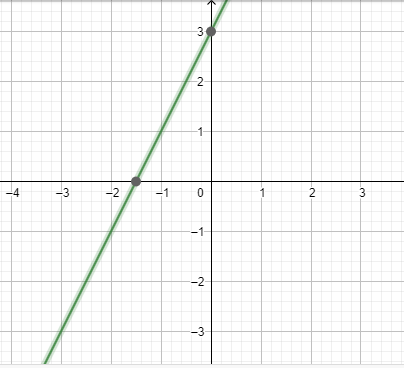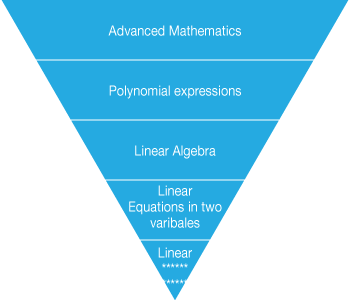In the verge of coronavirus pandemic, we are providing FREE access to our entire Online Curriculum to ensure Learning Doesn't STOP!

# One-Variable Linear Equations and Inequations

Go back to  'Linear and Quadratic Equations'

Introduction: One Variable Linear Equations and Inequations. You may not believe it but linear equations and inequations are an everyday part of our lives. Let us suppose that a factory produces logs that are always approximately 10 m long. The machine is not precise enough to produce logs that are exactly 10 m long, however the logs are never, ever longer than 10 m. So, this would create an inequation of the nature:

$$x < 10$$

## The Big Idea: What are One variable linear equations and inequations?

An algebraic equation is a statement that equates two mathematical expressions.

$$x + 5 = 4;$$

$$2x + 9 = 23$$

Any mathematical equation or inequation has 2 sides to it. Left Hand Side (LHS) and Right Hand Side (RHS). In case of an equation, the 2 sides are equal, that is, Left Hand Side is equal to Right Hand side. For example, 2 plus 4 is equal to six is an equation expressed in words. The best way to visualise equations and inequations is to picture weighing balances.

Here is an example of what an equation looks like, where the RHS = LHS.On the other hand, inequations represent inequalities between the Left Hand side and the Right Hand Side.

Here is an example of an inequation where, the RHS =/= LHS. In the below illustration we can see that the expression on the left hand side, that is, $${3x - 4}$$, is in fact greater than the number on the right hand side, that is 20. Hence, we may express the inequality as: $${3x-4 > 20}$$. We use the notation (>) for representing greater than, while (<) notation is used for an expression of lesser than.A Linear equation is an equation where the power of the variable is 1. This means that the variables in a linear equation do not have exponents like squares or cubes. What do we mean when we say, “one variable linear equation”?  Well, It has a single variable (unknown); it is linear i.e. the pattern it makes is a straight line and not a parabola or any non-straight curve and it is an equation which means that LHS = RHS. In case of an inequation, instead of equality, some other relationship like less than or greater than exists between LHS and RHS.($$y = 2x + 3;$$ linear equation is plotted as a straight line)

## Why One Variable linear equations and inequations? Why are they important?

Writing problem statements as equations gives one the flexibility to convey complex mathematical ideas as simpler expressions. This is what makes equations one of the most essential tools in your math kit, and therefore imperative that you master them. Linear equations and inequations in one variable are the entry point to one of the most powerful mathematical tools around.One of the most common misconceptions that students have is that they think that the variable is just a substitute for a particular number. That is NOT the case. As the term suggests, the variable can have multiple values that satisfy the condition that the algebraic expression describes.

An important point to note while attempting to solve linear equations or inequations is that you should try to get the variable to one side of the equation (usually on the left side, LHS) while keeping the values or numbers to the right side. This will help you get the solution of the equation. Another extremely important rule of equations or inequations is that you should always perform the same operation on both the sides of equality or inequality, that is, if you are adding or subtracting the LHS (Left Hand Side) with a particular number, make sure that you add or subtract the RHS (Right Hand Side) with the same particular number.

## Tips and Tricks

• Always visualise one variable linear equations as straight lines. Build your intuition around them. Just plot two points and you have figured out the entire straight line. You’ll know how steep it is and its general orientation on the coordinate axis.
• All one variable linear equations will be of the form:
5x + 2 = 0 where x is the variable.
The coefficients and constant will be different for different equations.
The coefficient of the x term (5 in this example) tells you how steep the line is. Larger the coefficient of x, the steeper the line. And the constant (2 in our example) tell you how far from the origin does the line pass. Here 2 indicates that when crossing the Y-axis the line will be 2 units above the origin.
One-Variable Linear Equations and Inequations